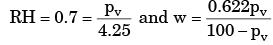Courses

# Past Year Questions: Properties Of Moist Air

## 12 Questions MCQ Test Engineering Mechanics | Past Year Questions: Properties Of Moist Air

Description
This mock test of Past Year Questions: Properties Of Moist Air for Mechanical Engineering helps you for every Mechanical Engineering entrance exam. This contains 12 Multiple Choice Questions for Mechanical Engineering Past Year Questions: Properties Of Moist Air (mcq) to study with solutions a complete question bank. The solved questions answers in this Past Year Questions: Properties Of Moist Air quiz give you a good mix of easy questions and tough questions. Mechanical Engineering students definitely take this Past Year Questions: Properties Of Moist Air exercise for a better result in the exam. You can find other Past Year Questions: Properties Of Moist Air extra questions, long questions & short questions for Mechanical Engineering on EduRev as well by searching above.
QUESTION: 1

### Atmospheric air from 40°C and 60 percent relative humidity can be brought to 20°C and 60 percent relative humidity by 

Solution:

Cooling and dehumidification process,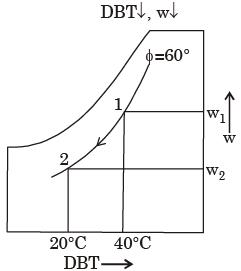QUESTION: 2

### If moist air is cooled by sensible heat removal, which of the following is true? 

Solution:

Sensible cooling process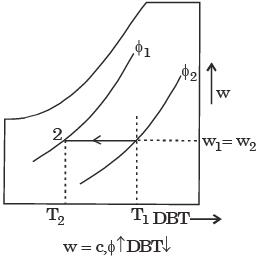QUESTION: 3

### For air at a given temperature, as the relative humidity is increased isothermally, 

Solution: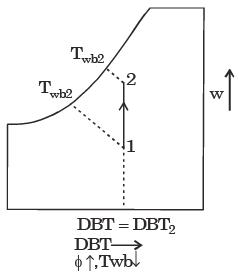Relative humidity is increased isothermally.

QUESTION: 4

For air with a relative humidity of 80%



Solution: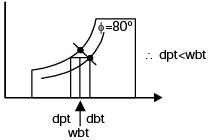QUESTION: 5

Dew point temperature of air at one atmospheric pressure (1.013 bar) is 18°C. The air dry bulb temperature is 30°C. The saturation pressure of water at 18°C and 30°C are 0.02062 bar and 0.04241 bar respectively.
The specific heat of air and water vapour respeetively are 1.005 and 1.88 kJ/kgK and the latent heat of vaporization water of 0°C is 2500 kJ/kg. The specific humidity (kg/kg of dry air) and enthalpy (kJ/kg of dry air) of this moist air respectively, are



Solution:

Given: p = 1.013 bar, (pv)tdp = 0.02062
Specific humidity =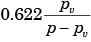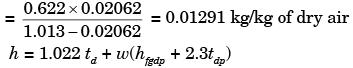= 63.15 kJ/kg of dry air.
Alternative method
pv = Saturation pressure = 0.02062 bar pa = Partial pressure of air = 0.04241 bar
td = Dry bulb temperature = 30º
tw = Wet bulb temperature = 18º Specific humidity,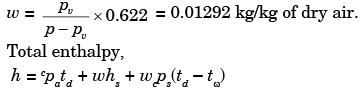= 1.005 × 30 + 0.0129 × 2500
+ 0.01292 × 1.88(30 – 18)
= 63.15 kJ/kg of dry air.

QUESTION: 6

For a typical sample of ambient air (at 30°C, 75% relative humidity and standard atmospheric pressure), the amount of moisture in kg per kg of dry air will be approximately.



Solution:

Given data:
Dry bulb temperature:
Tdb = 35°C
Relative humidity
φ = 75° = 0.75
At 35°C, Ps = .05628 bar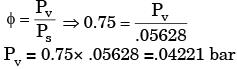Specific humidity,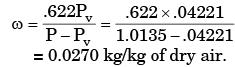QUESTION: 7

A building has to be maintained at 21 °C (dry bulb) and 14.5°C(wet bulb). The dew point temperature under these conditions is 10.17°C.The outside temperature is –23°C (dry bulb) and the internal and external surface heat transfer coefficients are 8 W/m2K and 23 W/ m2K respectively. If the building wall has a thermal conductivity of 1.2 W/mK, the minimum thickness (in m) of the wall required to prevent condensation is



Solution:

Given data:
Dry bulb temperature:
Tdb = 35°C
Relative humidity
φ = 75° = 0.75
At 35°C, Ps = .05628 barSpecific humidity,QUESTION: 8

Moist air at a pressure of 100 kPa is compressed to 500 kPa and then cooled to 35°C in an aftercooler. The air at the entry to the aftercooler is unsaturated and becomes just saturated at the exit of the aftercooler. The saturation pressure of water at 35°C is 5.628 kPa. The partial pressure of water vapour (in kPa) in the moist air entering the compressor is closest to



Solution:

Assuming that compression is isentropicin air compressors, the process can be described on the T - s diagram. The process in the intercooler is constant pressure
p2 = p3
But p2 = 5p1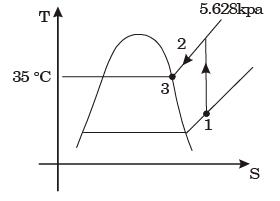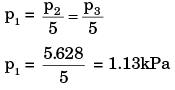QUESTION: 9

A moist air sample has dry bulb temperature of 30°C and specific humidity of 11.5 g water vapour per kg dry air. Assume molecular weight of air as 28.93. If the saturation vapour pressure of water at 30°C is 4.24 kPa and the total pressure is 90 kPa, then the relative humidity (in %) of air sample is



Solution:

Given : DBT = 30°C, w = 11.5 g/kg
∴ Relative humidity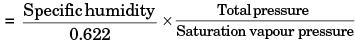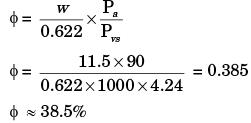QUESTION: 10

If a mass of moist air in an airtight vessel is heated to a higher temperature, then



Solution:

R.H . decreases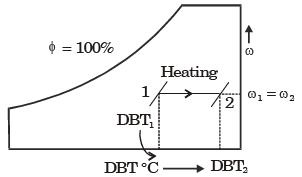QUESTION: 11

A room contains 35 kg of dry air and 0.5 kg of water vapor. The total pressure and temperature of air in the room are 100 kPa and 25°C respectively. Given that the saturation pressure for water at 25°C, is 3.17 kPa, the relative humidity of the air in the room is



Solution:

ma = 35 kg, mV = 0.5 kg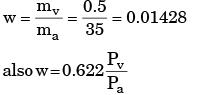Pt = 100 kPa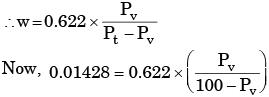PV = 2.238 kPa
Relative humidity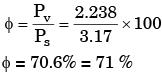QUESTION: 12

The pressure, dry bulb temperature and relative humidity of air in a room are 1 bar, 30°C and 70%, respectively. If the saturated steam pressure at 30°C is 4.25 kPa, the specific humidity of the room air in kg of water vapour/kg dry air is



Solution: